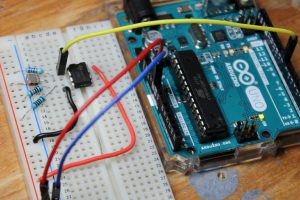# Use a Comparator or Op-Amp to Simplify Light Dependent Resistor Output

If your project calls for light sensitivity, it’s hard to beat light dependent resistors (LDRs), also known as photoresistors. They’re available for a few cents each, and their resistance varies based on how much light they receive. In the dark, these devices produce resistances in the megohm range, and can fall to hundreds of ohms or even less when exposed to sufficient light. You first instinct when prototyping this type of device is likely to use an analog input on an Arduino or similar dev board to sense voltage levels. This works quite well in many situations, but you may also want to consider a comparator or operational amplifier (op-amp) to turn this analog input into a simple on/off signal. You could also use one of these components by itself to produce a usable output without the use of a microcontroller.First, let’s examine how a microcontroller would see an LDR input. Using the circuit illustrated in the figure above with an Arduino Uno, an LDR is attached to 5VDC, then routed to the analog input A0. Voltage at the intersection of A0, the resistor, and LDR is divided between the fixed resistor and LDR, which decreases its resistance as light is applied. Voltage at this analog input increases with the lowered resistance in proportion to the amount of light the LDR sees.

The Arduino board is thus able to sense the resulting voltage level and convert it to an analog value. A threshold can be setup to respond to different light levels as on or off, or the analog signal can be used for proportional control. Note that the resistor in this illustration is just a placeholder; it would need to be adjusted based on your LDR sensitivity. You can also use a trimming resistor to tweak output values as needed.

Comparator Digitizes Analog Signal

What if you need light input, but just want an on/off value? Analog inputs can handle this programmatically, but if you’re using an Arduino Uno you’re restricted to the 6 analog pins. There’s also the normally minor issue of additional program complexity. If you need more performance out of your setup, you could turn to a comparator, or operational amplifier (op-amp) set up to act as one, to convert this analog value into a simple on/off signal.

Read more: Use a Comparator or Op-Amp to Simplify Light Dependent Resistor Output